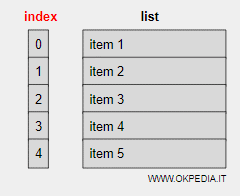# How to make a list in Python

To create a list in the python language, use the square brackets in the assignment operation.

list = [ item1, item2, ... ]

The elements in brackets are separated by commas and can also be of different types.

Each element is assigned an index starting from zero that identifies it.

Note. The first element (item 1) of a list is associated to the index zero, the second element to the index one, etc.## Practical examples of lists in Python

Example 1

This statement creates a list called colors.

color = [ "red", "green", "yellow" ]

The elements of the list are three alphanumeric strings, each indicating a different color.

The indexes of the list are the following

colori="red"
colori="green"
colori="yellow"

Example 2 ( how to access an item )

To print only the second item in the list

print(color)

The output result is

green

Example 3 (mixed lists)

A list can also contain values of different types.

year = [ "millennium", 2001, 2002 ]

This list contains a string and two numeric values.

Example 4 (list of lists)

A list can also contain other lists.

list = [1,2,3, ["A","B","C"] ]

The elements of the list are

list=1
list=2
list=3
list=["A","B","C"]

The fourth element of the main list is another list.

https://how.okpedia.org/en/python/how-to-make-a-list-in-pythonReport us an error or send a suggestion to improve this page

Python List